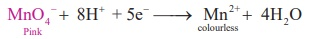Home | | Chemistry 12th Std | Estimation of oxalic acid

# Estimation of oxalic acid

To estimate the amount of oxalic acid dissolved in 500 ml of the given solution volumetrically.

Estimation of oxalic acid

## Aim :

To estimate the amount of oxalic acid dissolved in 500 ml of the given solution volumetrically. For this you are given with a standard solution of ferrous ammonium sulphate (FAS) of normality 0.1 N and potassium permanganate solution as link solution.

## Principle:

During these titrations, oxalic acid is oxidized to CO2 and MnO4- ions (from KMnO4 ) is reduced to Mn2+ ion.

Oxidation  : MnO + 8H+  + 5e       Mn2+ + 4HReduction :Overall reaction

5(COOH)2 + 2MnO4 + 6H+     → 10CO2 + 2Mn2+ + 8H2O

Since one mole oxalic acid releases 2 moles of electrons, the equivalent weight of oxalic acid = 106/2 = 63 (oxalic acid is dihydrated)

### Short procedure:## Procedure :

### Titration–I

Burette is washed with water, rinsed with KMnO4 solution and filled with same KMnO4 solution up to the zero mark. Exactly 20 ml of standard FAS solution is pipetted out into the clean, washed conical flask. To this FAS solution, approximately 20ml of 2N sulphuric acid is added. This mixture is titrated against KMnO4 Link solution from the burette. KMnO4 is added drop wise till the appearance of permanent pale pink colour. Burette reading is noted and the same procedure is repeated to get concordant values.

### Titration –I

(Link KMnO4 )Vs (Standard FAS solution)## Calculation :

Volume of KMnO4 (link) solution V1 = ml

Normality KMnO4 (link) solution N1 = ? N

Volume of standard FAS solution V2 = 20 ml

Normality of standard FAS solution N2 = 0.1 N

According to normality equation:

V1 x N1 = V2 x N2

N1 = V2 x N2 / V1  =Normality KMnO4 (link) solution N1 = ____________ N

### Titration–II

(Unknown oxalic acid ) Vs (Link KMnO4 )

Burette is washed with water, rinsed with KMnO4 solution and filled with same KMnO4 solution up to the zero mark. Exactly 20 ml of unknown oxalic acid solution is pipetted out into the clean, washed conical flask. To this oxalic acid solution approximately 20ml of 2N sulphuric acid is added. This mixture is heated to 60 – 700C using Bunsen burner and that hot solution is titrated against KMnO4 Link solution from the burette. KMnO4 is added drop wise till the appearance of permanent pale pink colour. Burette reading are noted, the same procedure is repeated to get concordant values.

### Titration –II

(Link KMnO4 )Vs (Unknown oxalic acid)## Calculation :

Volume of Unknown oxalic acid solution V1 = 20 ml

Normality of Unknown oxalic acid solution N1 = ? N

Volume of KMnO4 (link) solution V2 = ml

Normality KMnO4 (link) solution N2 = N

According to normality equation:

V1 x N1 = V2 x N2

N1 = V2 x N2 /  V1Normality of Unknown oxalic acid solution N1 = ________ Y _______ N

## Weight calculation:

The amount of oxalic acid dissolved in 1 lit of the solution  =(Normality) x (equivalent weight)

The amount of oxalic acid dissolved in 500 ml of the solution  = [Y × 63 × 500] /  1000

=  [ ? x 63 x 500] / 1000

= ____ g

## Report :

The amount of oxalic acid dissolved in 500 ml of given the solution = g

Tags : Volumetric Analysis | Chemistry Practical Laboratory Experiment , 12th Chemistry : Practicals
Study Material, Lecturing Notes, Assignment, Reference, Wiki description explanation, brief detail
12th Chemistry : Practicals : Estimation of oxalic acid | Volumetric Analysis | Chemistry Practical Laboratory Experiment